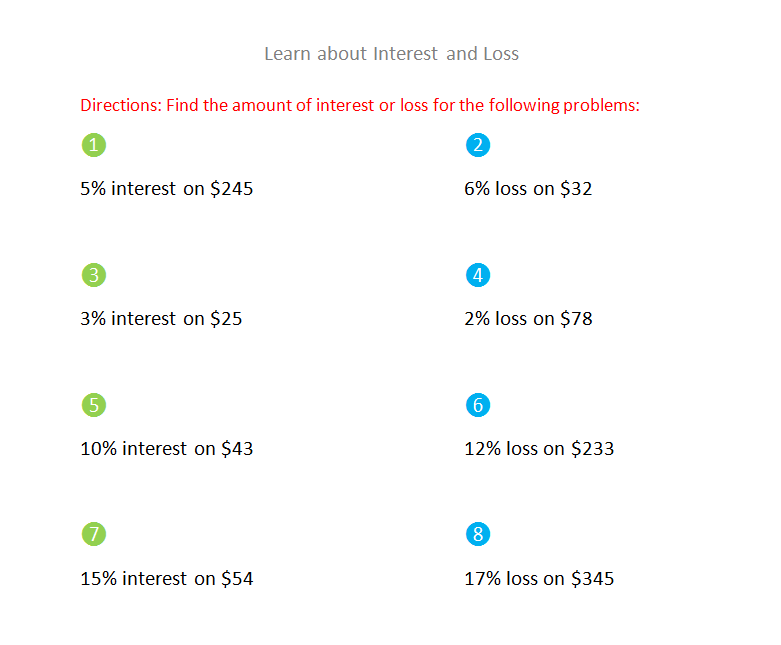## Preview

What is interest and loss?
Well that is a very good question because this topic is really good to know about because it is very influential in life.

Well the first thing you need to know is that both interest and loss have to deal with money, so first let's talk about interest.
Interest is the additional money on the borrowed money. Like for example if you have borrowed \$200 from someone and the someone says that the interest is 5% so that means its \$200 x 5% = \$40 so the amount of interest that you will give is \$40.

Now lets talk about loss, and loss is something that you loose like you buy a ball for \$45 and sell it to someone for \$40 then the amount of loss will be \$5 and so what we have to do is multiply 100% by the loss over the original cost price like this:
\$5   x 100% = 11 approx.
\$45

So now that you know about interest and loss you have studied a part of investment and if you like this topic then go ahead and learn more about investment. Now practice this and make sure to watch the video on YouTube.

##Sheet 1 Learn about Interest and Loss